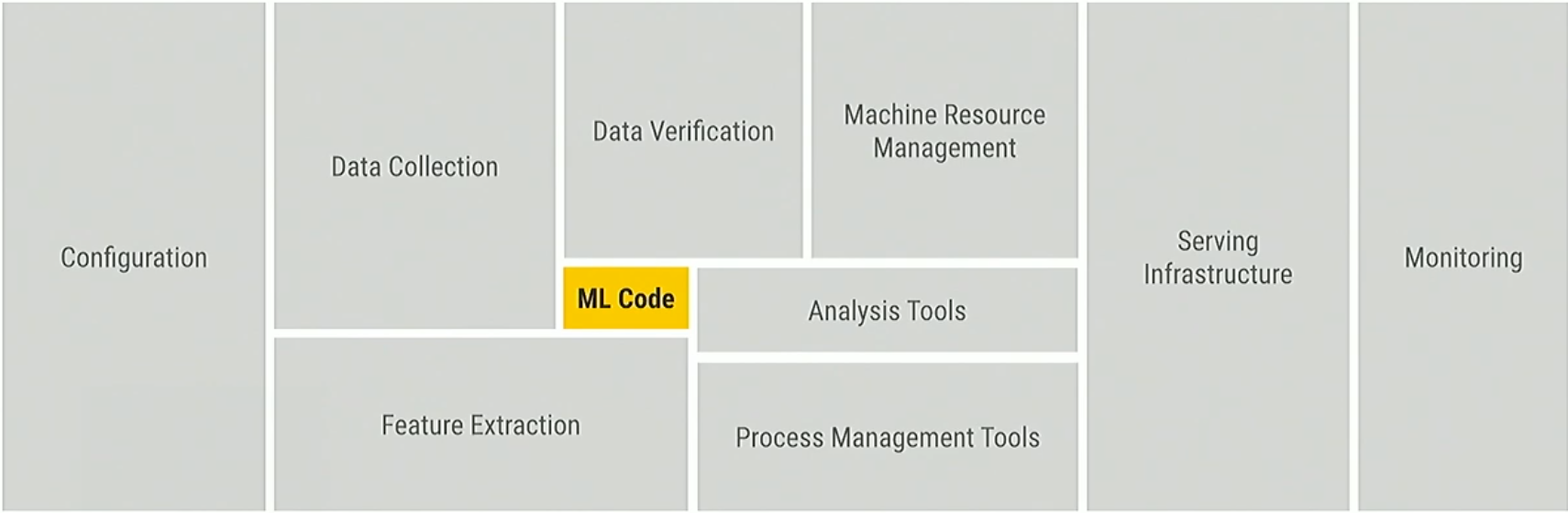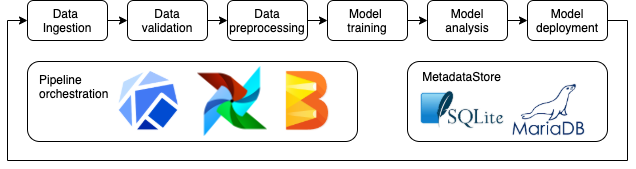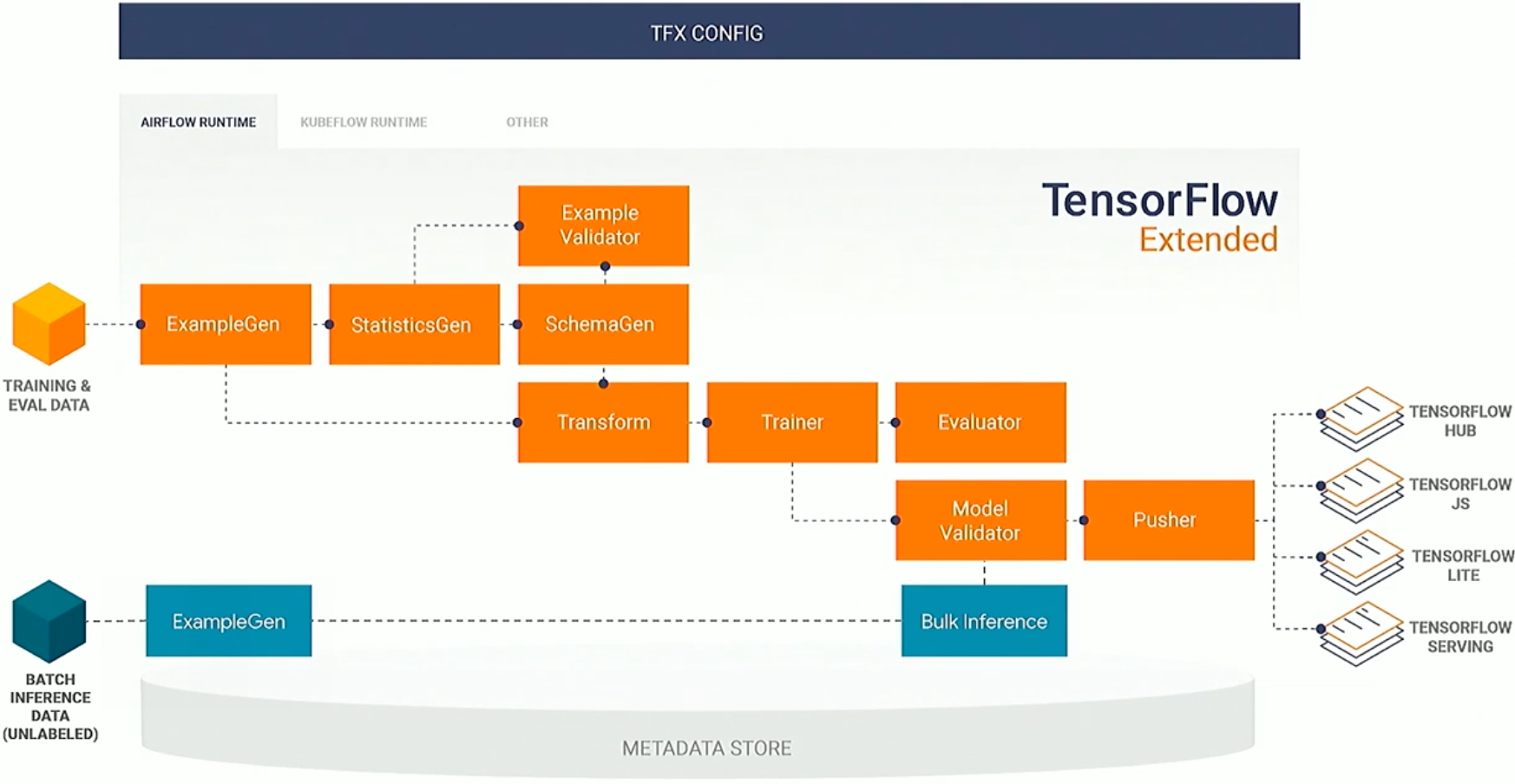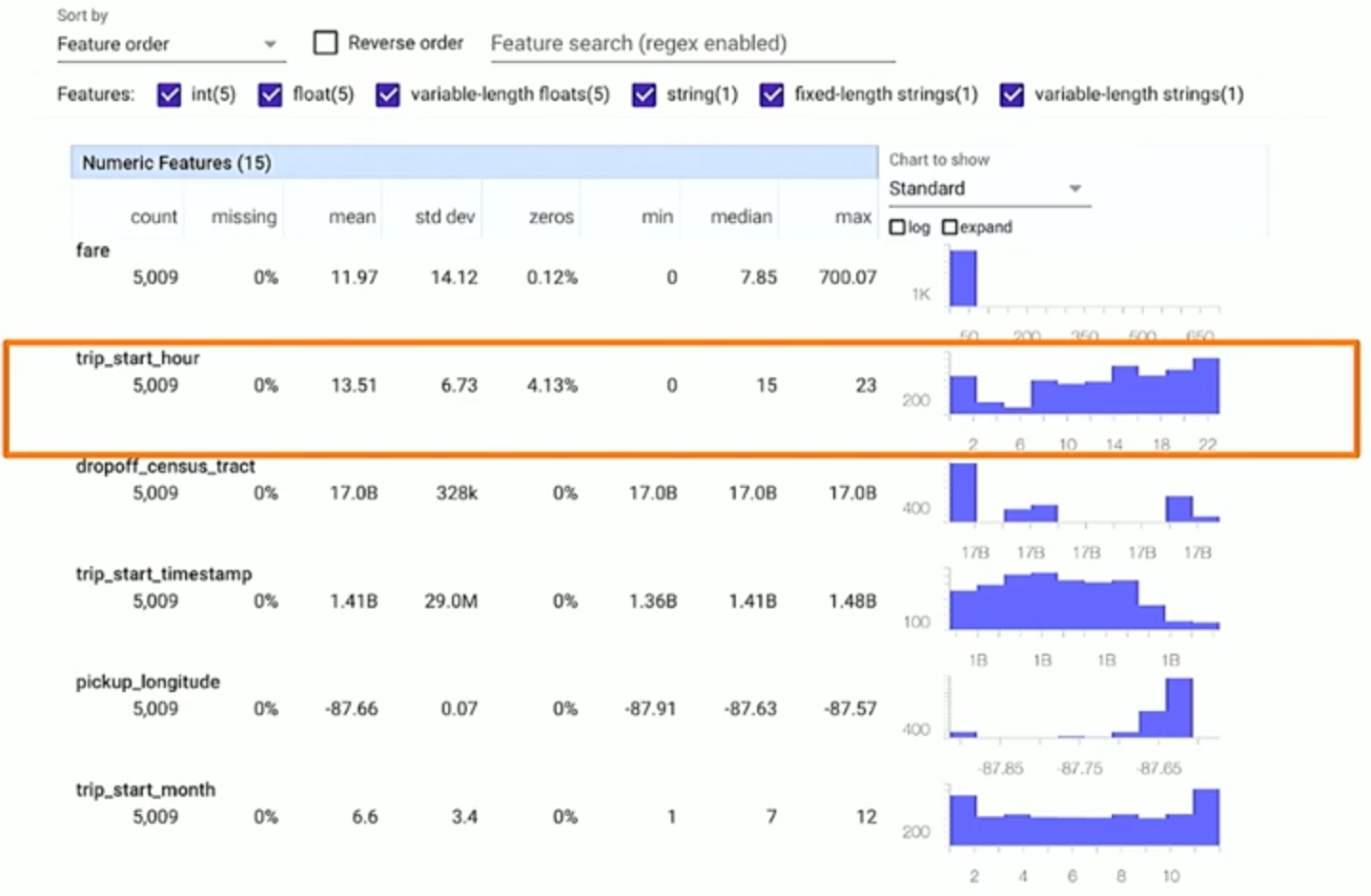# Demystifying TFX Standard ComponentsWhen we think about ML we tend to focus on the model training part. But when we move to production we realize that there many other pieces that are very important for the model to be available and robust over its lifetime.

A production solution requires so much more to be able to deal with all issues that we face during ML development:

• Labeled data (how to get it) I may have terabytes of data but I need a label for them
• Feature space coverage: does my data cover the feature space when I’m going to run inference on them
• Minimal dimensionality: is my dimensionality minimized or can I do more to simplify my feature set/vector to make the model more efficient
• Maximum predictive data: how to get the predictive information in the data I’m choosing
• Fairness: Are we serving all customers fairly no matter where they are, what religion, what language they speak, what demographic they may be. You want to serve those people as well as you can, you don’t want disadvantaged people.
• Rare conditions: especially in things like healthcare, we make a prediction that’s very important to someone’s life on a condition that occurs very rarely
• Data lifecycle management: understanding this is important, once you have trained a model and put it in production this is the starting point, how are we going to maintain that over the lifetime as the world changes, data changes, things in your domain changes.

## Introduction

TFX is a flexible ML platform that lets users build ML pipelines, using different orchestrators and different underlying execution engines. It also implements some best practices to standardize ML models lifecycle management:

• Python-based classes for components definition
• Strongly-typed artifacts (i.e. components input/output)
• Metadata storage backed by MySQL for artifact and execution tracking
• Pipeline configuration through text editors or with notebooks
• Workflow execution supported by common OSS orchestrators: Apache Airflow, Apache Beam, Kubeflow
• Extensibility and portability
• and moreConceptually, TFX has a layered architecture to coordinate the execution of its components. The layers are:

• Metadata storage: storage for artifacts produced by the components, it enables performing comparison across months/years and see how things change
• Job orchestration: responsible for orchestrating the execution of the flow of components in a TFX pipeline.
• Configuration framework: powers the configuration of TFX components

## Terminology

At the heart of TFX is the Metadata Store which is responsible for containing:

• Strongly typed definitions of artifacts (trained models, datasets, or other objects) and their properties
• Execution records of component and pipeline run
• Workflow provenance across all executions
• Grouping of artifact and execution records (e.g. Pipeline Run, Experiment Session)

### Components

A TFX component is responsible for performing a specific task, for instance, data ingestion, model training with TensorFlow, or serving with TF Serving. Every component in TFX has three building blocks:

• A driver consumes artifact and the execution of the component
• A publisher takes the output of the component and put it back to the Metadata Store.
• An Executor is where the work is done and is the part that you can change, take an existent component overrides it to create a new.

### Pipeline

When TFX components are connected they form a pipeline through which data will flow, e.g. from ingestion data to serving models. The communication happens over the metadata store, each component read its dependencies from it and write back its output/artifact.

## Standard Components

There is a set of standard components which are shipped with TFX, which we can build/extend upon them in a couple of different ways.At the left we ingest data, we flow through, calculate some statistics about it, then we make sure there is no problem with the data, understand what type of feature we have, do feature engineering, we train, check the metrics, and then the question should I push this new model to production (if the new model outperforms existent one). Along with that we also have the ability to do bulk inference.

### ExampleGen

This component takes raw data as input and generates TensorFlow examples, it can take many input formats (e.g. CSV, TF Record). It also does split the examples for you into Train/Eval. It then passes the result to the StatisticsGen component.

examples = csv_input(os.path.join(data_root, 'simple'))
example_gen = CsvExampleGen(input_base=examples)


### StatisticsGen

StatisticsGen generates useful statistics that help diving into the data and understanding its characteristics. It also comes with visualization tools.

For instance, in the following example the column trip_start_hour seems to have a time window between 5 am and 6 am where data is missing. Such a histogram helps determine the area we need to focus on to fix any data-related problems. In this we need to get more data, otherwise, the inference for 6 am data will be overgeneralized.statistics_gen = StatisticsGen(input_data=example_gen.outputs.examples)


### SchemaGen

SchemaGen is looking at the data type of the input, is it an int, float, categorical, etc. If it is categorical then what are the valid values? It also comes with a visualization tool to review the inferred schema and fix any issues.

infer_schema = SchemaGen(stats=statistics_gen.outputs.output)


### ExampleValidator

ExampleValidator takes the inputs and looks for problems in the data (missing values, 0 values that should not be 0) and report any anomalies.

validate_stats = ExampleValidator(
stats=statistics_gen.outputs.output,
schema=infer_schema.outputs.output
)


### Transform

Transform takes data generated by the ExampleGen component and the schema generated by the SchemaGen to implement arbitrary complex logic, depending on the need of the dataset and model, e.g. to perform features engineering.

Note that the logic within this component cannot be eagerly executed as it will be turned into a graph that will be prepended to the model. This means that we will be doing the same feature engineering with the same code during both training and production which eliminates the training-serving skew.

transform = Transform(
input_data=example_gen.outputs.examples,
schema=infer_schema.outputs.output,
module_file=taxi_module_file
)
# do some transformation
for key in _DENSE_FLOAT_FEATURE_KEYS:
outputs[_transformed_name(key)] = transform.scale_to_z_score(_fill_in_missing(inputs[key]))
# ...
outputs[_transformed_name(_LABEL_KEY)] = tf.where(
tf.is_nan(taxi_fare),
tf.cast(tf.zeros_like(taxi_fare), tf.int64),
# Test if the tip was > 20% of the fare
tf.cast(tf.greater(tips, tf.multiply(taxi_fare, tf.constant(0.2))), tf.int64)
)
# ...


### Trainer

Trainer performs the training of the model. It uses TensorBoard to log performance metrics which helps to understand the training process and comparing execution runs.

trainer = Trainer(module_file=taxi_module_file,
transformed_examples=transform.outputs.transformed_examples,
schema=infer_schema.outputs.output,
transform_output=transform.outputs.transform_output,
train_steps=10000, eval_steps=5000, warm_starting=True
)


### Evaluator

Evaluator is a tool that lets us not only looking at top-level metrics (RMSE, AUC) but also looking at individual slices of the dataset and slices of features within the dataset. Things like Fairness becomes very manageable with this component.

model_analyzer = Evaluator(
examples=examples_gen.outputs.output,
eval_spec=taxi_eval_spec,
model_exports=trainer.outputs.output
)


### ModelValidator

This component helps to compare the different version of a model, e.g. a production model against a new model which is in current development using different validation modes:

• Validate using current eval data
• “Next-day eval”, validate using unseen data
model_validator = ModelValidator(
examples=examples_gen.outputs.output,
model=trainer.outputs.output,
eval_spec=taxi_mv_spec
)


### Pusher

This component is responsible for pushing the trained (and validated) model different deployment options:

• Filesystem (TensorFlow Lite, TensorFlow JS)
• TensorFlow Serving

It can be configured to block deployment on outcome of model validation.

pusher = Pusher(
model_export=trainer.outputs.output,
model_blessing=model_validator.outputs.blessing,
serving_model_dir=serving_model_dir
)


### BulkInferrer

BulkInferrer performs offline batch inference over inference examples. It outputs the features and predictions of the model.

It can be configured to block the inference on a model validation outcome. AlsoL

• Choose the inference examples from example gen’s output.
• Choose the signatures and tags of the inference model.
bulk_inferrer = BulkInferrer(
examples=inference_example_gen.outputs['examples'],
model_export=trainer.outputs['output'],
model_blessing=model_validator.outputs['blessing'],
data_spec=bulk_inferrer_pb2.DataSpec(example_splits=['unlabelled']),
model_spec=bulk_inferrer_pb2.ModelSpec()
)
context.run(bulk_inferrer)


## TFX Pipeline

The previous standard components can be used together to create a pipeline. The following code snippet illustrates how to create a TFX pipeline:

• Define the components, their input, and output
• Create a runner (e.g. Airflow) that will execute the pipeline
• Pass the list of components to the runner to initiate the pipeline execution

Here is a concrete example:

def _create_pipeline():
"""Implements a TFX pipeline."""
csv_data = csv_input(os.path.join(data_root, 'simple'))
example_gen = CsvExampleGen(input=csv_data)

statistics_gen = StatisticsGen(examples=example_gen.outputs['examples'])
infer_schema = SchemaGen(statistics=statistics_gen.outputs['statistics'])
validate_stats = ExampleValidator(statistics=statistics_gen.output['statistics'], schema=infer_schema.outputs['schema'])

# Performs feature engineering
transform = Transform(examples=example_gen.outputs['examples'], schema=infer_schema.outputs['schema'], module_file=_taxi_module_file)

trainer = Trainer(...)
model_analyzer = Evaluator(examples=example_gen.outputs['examples'], model=trainer.outputs['model'])
model_validator = ModelValidator(examples=example_gen.outputs['examples'], model=trainer.outputs['model'])
pusher = Pusher(model=..., model_blessing=..., serving_model_dir=...)

return [example_gen, statistics_gen, infer_schema, validate_stats, transform, trainer, model_analyzer, model_validator, pusher]

result = AirflowDAGRunner(_airflow_config).run(_create_pipeline())